Courses

# Competition Level: Electrostatics- 1

## 30 Questions MCQ Test Physics Class 12 | Competition Level: Electrostatics- 1

Description
This mock test of Competition Level: Electrostatics- 1 for Class 12 helps you for every Class 12 entrance exam. This contains 30 Multiple Choice Questions for Class 12 Competition Level: Electrostatics- 1 (mcq) to study with solutions a complete question bank. The solved questions answers in this Competition Level: Electrostatics- 1 quiz give you a good mix of easy questions and tough questions. Class 12 students definitely take this Competition Level: Electrostatics- 1 exercise for a better result in the exam. You can find other Competition Level: Electrostatics- 1 extra questions, long questions & short questions for Class 12 on EduRev as well by searching above.
QUESTION: 1

### One quantum of charge should be at least be equal to the charge in coulomb:

Solution:

Quantum of charge is represented by q=ne... where e is the charge of an electron. for 1 quantum of charge the value is 1.6 × 10-19C

QUESTION: 2

### The force between two charges is 120 N. If the distance between the charges is doubled, the force will be

Solution: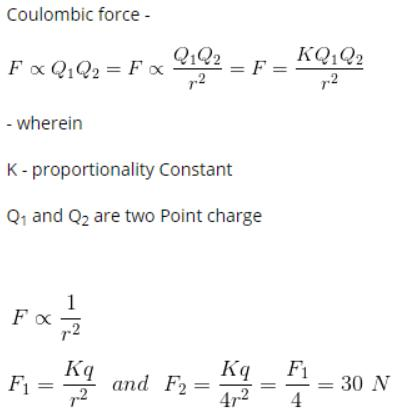QUESTION: 3

### Which one of the following statement regarding electrostatics is wrong ?

Solution:

A stationary charge doesn't produce a magnetic field , it only produces an electric field.

QUESTION: 4

Two similar charge of +Q , as shown in figure are placed at A and B. _q charge is placed at point C midway between A and B. _q charge will oscillate if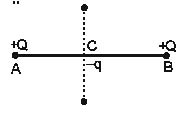Solution:

When -q is displaced left or right of the force is increased so -q move towards that force hence will not perform SHM but when its displaced to AB it will perform SHM because it will return to its mean position.

QUESTION: 5

When the distance between two charged particle is halved, the force between them becomes

Solution:

Earlier force between two particles is
F=kq1​q2​​/ r2
When distance is halved force becomes
F′= kq1​q2/(r/2)2 ​​=4kq1​q2/r2​​=4F

QUESTION: 6

Two point charges in air at a distance of 20 cm. from each other interact with a certain force. At what distance from each other should these charges be placed in oil of relative permittivity 5 to obtain the same force of interaction

Solution: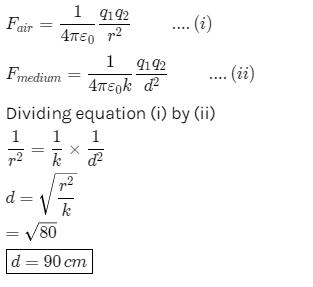QUESTION: 7

A certain charge Q is divided at first into two parts, (q) and (Q-q). Later on the charges are placed at a certain distance. If the force of interaction between the two charges is maximum then

Solution:

The force between q & Q-q is given by :-
F = kq(Q-q)/r²
As it is given that force between these two is Maximum....so it will be max. when dF/dq is zero
i.e, dF/dq = {k(Q-2q)}/r² = 0
so, Q = 2q
Q/q = 2/1

QUESTION: 8

Two small balls having equal positive charge Q (Coulomb) on each are suspended by two insulating strings of equal length 'L' metre, from a hook fixed to a stand. The whole set up is taken in a satellite in to space where there is no gravity (state of weight lessness). Then the angle (q) between the two strings is

Solution:

There is a condition of weightlessness in a satellite.
Therefore, mg=0
Due to electrostatic force of repulsion between the balls, the string would become horizontal.
Therefore, angle between the string =180

QUESTION: 9

Two point charges -1 × 10-6 C and 4 × 10-6 C separated by 3 m distance have zero field location of

Solution: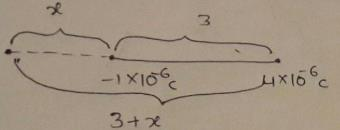x=l/[ √(q2/q1)-1]
=3/√[(4x10-6/1x10-6)-1]
= (3/2)-1
=3
So, 3m away from -1x10-6C

*Multiple options can be correct
QUESTION: 10

Two charges 4q and q are placed 30 cm. apart. At what point the value of electric field will be zero

Solution:

Where E=0 this point is called neutral point.
it is the point where electric field of both charge is same
we know tha t E=kQ/r^2
here k=1/4pi€
for 4q charge
let at ''a'' distance we get E=0 which is from q charge
so distance from 4q of 'a' point is 30-a
electric field by 4q charge on a is
E=k4q/(30-a)^2
electric field by q charge on a point
E=kq/a^2
both electric field are equal so put them equal
k4q/(30-a)^2=kq/a^2
solve this we get
4a^2=(30-a)^2
2a=30-a
3a=30
a=10
so at a distance 10cm from charge q we get E=0
distance from 4q charge 30-10=20cm.

QUESTION: 11

If Q =2 coloumb and force on it is F=100 newtons , Then the value of field intensity will be

Solution:

Electric force on a charge q placed in a region o electric field intensity is E and it is given by F = qE.
In this case, F = 100 N and q = 2 C.
So, E=F/q​=100N/2C​=50 N/C.
Hence, the value of field intensity will be 50 N/C.

QUESTION: 12

Four equal but like charge are placed at four corners of a square. The electric field intensity at the center of the square due to any one charge is E, then the resultant electric field intensity at centre of square will be

Solution:

As the components of electric field intensity at diagonal are equal in magnitude and opposite in direction, thus the intensity of electric field will be zero.
Hence the correct answer would be option A.

QUESTION: 13

If mass of the electron = 9.1 × 10-31 Kg. Charge on the electron = 1.6 × 10-19coulomb and g = 9.8 m/s2. Then the intensity of the electric field required to balance the weight of an electron is

Solution:

We know that, qE=mg
So,E=mg/q
E=9.1x10-31x9.8x1019/1.6
E=9.1x9.8x10-12/1.6
E=5.6x10-11

QUESTION: 14

The electric field intensity at a point situated 4 meters from a point charge is 200 N/C. If the distance is reduced to 2 meters, the field intensity will be

Solution:

Field intensity is proportional to the inverse of the square of the distance separating the point charges:

F = k*q1*q2/d^2

Since k, q1, and q2 are assumed to be constant, their product can be combined to form a single constant:

K = k*q1*q2, so

F = K/d^2 (and by algebraic manipulation we have K=F*d^2).

Therefore we know that

F(at d=4) = K/4^2, and

F(at d=2) = K/2^2.

Since K is a constant, we can equate K=F*d^2 for each case:

K=F(at d=4)*4^2 = F(at d=2)*2^2

so

F(at d=2) = (F(at d=4)*4^2)/2^2

F(at d=2) = 200N/C * (4^2)/(2^2) = 200N/C *16/4 = 800N/C.

QUESTION: 15

At position of (4i + 3j) by a point charge 5 × 10-6 C placed at origin will produce electric field of

Solution: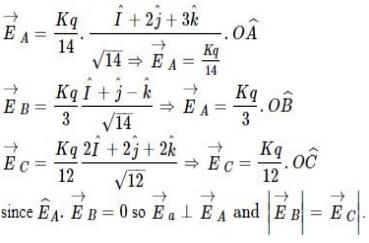QUESTION: 16

Two identical point charges are placed at a separation of l.P is a point on the line joining the charges, at a distance x from any one charge. The field at P is E. E is plotted against x for values of x from close to zero to slightly less than l. Which of the following best represents the resulting curve ?

Solution: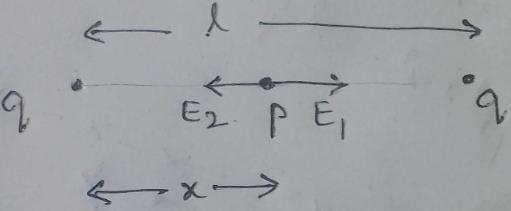At point P,
E=E1​−E2​ rightward
⟹E=(kq/x2​)−(kq/(l−x)2​)   where k=1/4πϵo​
⟹E=kq (l(l−2x)​/x2(l−x)2
From graph we can see that it can't be straight line and E=0 at x=l/2​
At x=0 ,,E→∞  and at x=l, E→−∞
Hence, from the shown graphs the correct answer is (D).

QUESTION: 17

A particle of mass m and charge Q is placed in an electric field E which varies with time t ass E = E0 sinwt. It will undergo simple harmonic motion of amplitude

Solution:

Due to verifying electric field, it experiences an verifying force :-
F=QE=QE0​sinωt
at maximum amplitude A, it experience a maximum force of:-
Fmax​=QE0​
also, Restoring force in SHM is given by: - F=mω2x
for amplitude, x=A OR,
2A=QE0​
⇒A= QE0/mω2

QUESTION: 18

Four charges are arranged at the corners of a square ABCD, as shown. The force on +ve charge kept at the centre of the square is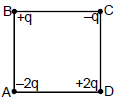Solution:
QUESTION: 19

Two free positive charges 4q and q are a distance l apart. What charge Q is needed to achieve equilibrium for the entire system and where should it be placed form charge q ?

Solution:

Let"r" be the distance from the charge q where Q is in equilibrium .Total Force acting on Charge q and 4q:
F=kqQ/r² + k4qQ/(l-r)²
For Q to be in equilibrium , F should be equated to zero.
kqQ/r² + k4qQ/(l-r)²=0
(l-r)²=4r²
⇒l-r=2r
⇒l=3r
⇒r=l/3
Taking the third charge to be -Q (say) and then on applying the condition of equilibrium on + q charge
kQ/(L/3)² =k(4q)/L²
9kQ/L²=4kq/L²
9Q=4q
Q=4q/9
Therefore a point charge -4q/9 should be placed at a distance of L/3 rightwards from the point charge +q on the line joining the 2 charges.

QUESTION: 20

Six charges are placed at the corner of a regular hexagon as shown. If an electron is placed at its centre O, force on it will be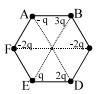Solution:

The charges will be balanced by their counterparts on the opposite side. So, eventually the charges remaining will be 2q and b and 3q on D.
Since the charge distribution is asymmetrical, the net force on charge would be skewed towards D.

QUESTION: 21

A charged particle of charge q and mass m is released from rest in an uniform electric field E. Neglecting the effect of gravity, the kinetic energy of the charged particle after time 't' seconds is

Solution:

Force on particle=F=qE
Hence, acceleration of particle=a=F/m​=qE/m​
Initial speed=u=0
Hence, final velocity=v=u+at=qEt/m​
Kinetic energy=K=(1/2)​mv2=(1/2)​m(qEt/m​)2
⟹K=E2q2t2​/2m

QUESTION: 22

Two identical positive charges are fixed on the y-axis, at equal distances from the origin O. A particle with a negative charge starts on the x-axis at a large distance from O, moves along the +x-axis, passes through O and moves far away from O. Its acceleration a is taken as positive along its direction of motion. The particle's acceleration a is plotted against its x-coordinate. Which of the following best represents the plot ?

Solution: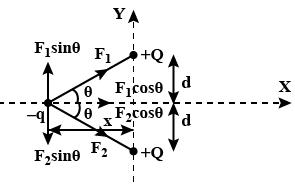Consider the attached free body diagram for the given scenario.
From coulomb's law,
∣F1​∣=∣F2​∣= qQ /4πεo​r2
In y-direction, forces cancel, Fy​=0
In x-direction, forces add up. Also, it is directed in +ve x direction if the position of −q is along −ve x axis and in −ve x direction if the position of −q is along +ve x axis.
Hence,
Fx​=2qQcosθ​ i /4πεo​r2    ∀ x<0
Fx​=2qQcosθ​ i /4πεo​r2    ∀ x≥0
But cosθ =x/r
r= √x2+d2​
Substituting in force equation, we get
Fx​=2qQx​ i /4πεo​( x2+d2​)3/2  ∀ x<0
Fx​=2qQx i /4πεo​( x2+d2​)3/2  ∀ x≥0

Now, a=F/m​ by Newton's second law. It is positive when x is negative and negative when x is positive. This is satisfied only in option (B).

QUESTION: 23

Four equal positive charges are fixed at the vertices of a square of side L. Z-axis is perpendicular to the plane of the square. The point z = 0 is the point where the diagonals of the square intersect each other. The plot of electric field due to the four charges, as one moves on the z-axis.

Solution:
QUESTION: 24

A small circular ring has a uniform charge distribution. On a far-off axial point distance x from the centre of the ring, the electric field is proportional to

Solution:

Electric field due to a charged ring in given by: -
at point p
∣​E∣​= KQx​ /(R2+x2)3/2        Q =λ(2πr)
at a large distance, x≫R, so :- R2+x2≃x2
⇒∣​E∣​= K&x​/(x2)3/2=KQ/x2​=Eαx−2
so at a large distance, the ring behaves as a point particle.

QUESTION: 25

A nonconducting ring of radius R has uniformly distributed positive charge Q. A small part of the ring, of length d, is removed (d<<R). The electric field at the centre of the ring will now be

Solution:

If we fill the gap then there will be no electric field at the center of ring
This signifies that electric field due to rest ring is equal to electric field due to gap and opposite in direction
so electric field due to gap= Kq/R2
q=Qd/2πR​
thus electric field E=KQd​/ R3
Hence E will be inversely proportional to R3  and direction will be toward the gap.

QUESTION: 26

The electric field intensity at a point situated 4 meters from a point charge is 200 N/C. If the distance is reduced to 2 meters, the field intensity will be

Solution:
QUESTION: 27

The direction (θ) of E at point P due to uniformly charged finite rod will be -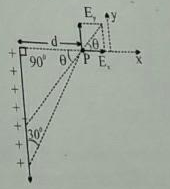Solution:

The angle suspended by the finite line charge at P=60°
So, the resultant electric field due to line charge will be at 60/2⇒30° since we can assume the charge concentrated at the centre of finite line charge.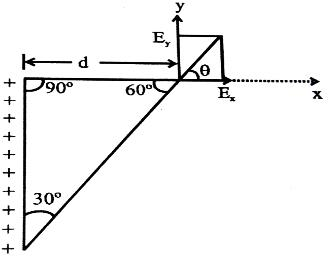QUESTION: 28

When charge of 3 coulomb is placed in a Uniform electric field , it experiences a force of 3000 newton, within this field, potential difference between two points separated by a distance of 1 cm is-

Solution:

Electric force F=qE
given, F=3000N,q=3C
∴E=F/q​=3000/3​=1000 V/m
Potential difference, V=Ed where d=0.01m
∴V=1000×0.01=10V

QUESTION: 29

An electric dipole is placed at an angle of 30° with an electric field intensity 2 × 105 N/C. It experiences a torque equal to 4 N m. The charge on the dipole, if the dipole length is 2 cm, is

Solution:
QUESTION: 30

Electric potential is a -

Solution:

Electric potential is a pure Scalar quantity, The reason is as follows.
The Electric Potential is defined as the amount of work-done per unit positive charge to bring from infinity to that point under the influence of the primary charge only.
U=W/q
And work done is defined as the dot product of force and displacement which is a scalar quantity.
W=F.S
Thus Electric potential is a scalar quantity.